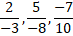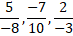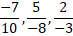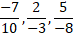# Quiz Discussion

When 0.232323..... is converted into a fraction, then the result is:

Course Name: Quantitative Aptitude

• 1]

1/5

• 2]

2/9

• 3]

23/99

• 4]

23/100

##### Solution
No Solution Present Yet

#### Top 5 Similar Quiz - Based On AI&ML

Quiz Recommendation System API Link - https://fresherbell-quiz-api.herokuapp.com/fresherbell_quiz_api

# Quiz
1
Discuss

5/9 of a number is equal to twenty five percent of second number. Second number is equal to 1/4 of third number. The value of third number is 2960. What is 30% of first number ?

• 1] 99.9
• 2] 88.8
• 3] 77.7
• 4] None of these
##### Solution
2
Discuss

The rational numbers lying between 1/3 and 3/4 are :

• 1]

$$\frac{{117}}{{300}},\frac{{287}}{{400}}$$

• 2]

$$\frac{{95}}{{300}},\frac{{301}}{{400}}$$

• 3]

$$\frac{{99}}{{300}},\frac{{301}}{{400}}$$

• 4]

$$\frac{{97}}{{300}},\frac{{299}}{{500}}$$

##### Solution
3
Discuss

The number 0.121212 ..... in the form p/q is equal to :

• 1]

2/11

• 2]

4/11

• 3]

2/33

• 4]

4/33

##### Solution
4
Discuss

$$\frac{{0.0203 \times 2.92}}{{0.0073 \times 14.5 \times 0.7}} = ?$$

• 1] 0.8
• 2] 1.45
• 3] 2.40
• 4] 3.25
##### Solution
5
Discuss

Given, 168 × 32 = 5376 , then 5.376 ÷ 16.8 is equal to :

• 1] 0.032
• 2] 0.32
• 3] 3.2
• 4] 32
##### Solution
6
Discuss

The price of commodity X increases by 40 paise every year, while the price of commodity Y increases by 15 paise every year. If in 2001, the price of commodity X was Rs. 4.20 and that of Y was Rs. 6.30, in which year commodity X will cost 40 paise more than the commodity Y ?

• 1] 2010
• 2] 2011
• 3] 2012
• 4] 2013
##### Solution
7
Discuss

The arrangement of rational numbers, -7/10, 5/-8, 2/-3  in ascending order is :

• 1]• 2]• 3]• 4]##### Solution
8
Discuss

Which part contains the fractions in ascending order ?

• 1]

$$\frac{{11}}{{14}},\frac{{16}}{{19}},\frac{{19}}{{21}}$$

• 2]

$$\frac{{16}}{{19}},\frac{{11}}{{14}},\frac{{19}}{{21}}$$

• 3]

$$\frac{{16}}{{19}},\frac{{19}}{{21}},\frac{{11}}{{14}}$$

• 4]

$$\frac{{19}}{{21}},\frac{{11}}{{14}},\frac{{16}}{{19}}$$

##### Solution
9
Discuss

Which of the following are in descending order of their value ?

• 1]

$$\frac{1}{3},\frac{2}{5},\frac{3}{7},\frac{4}{5},\frac{5}{6},\frac{6}{7}$$

• 2]

$$\frac{1}{3},\frac{2}{5},\frac{3}{5},\frac{4}{7},\frac{5}{6},\frac{6}{7}$$

• 3]

$$\frac{1}{3},\frac{2}{5},\frac{3}{5},\frac{4}{6},\frac{5}{7},\frac{6}{7}$$

• 4]

$$\frac{6}{7},\frac{5}{6},\frac{4}{5},\frac{3}{7},\frac{2}{5},\frac{1}{3}$$

##### Solution
10
Discuss

When 52416 is divided by 312, the quotient is 168. What will be the quotient when 52.416 is divided by 0.0168 ?

• 1] 3.12
• 2] 312
• 3] 3120
• 4] None of these
# Quiz